You may also likeConsecutive Numbers

An investigation involving adding and subtracting sets of consecutive numbers. Lots to find out, lots to explore.Calendar Capers

Choose any three by three square of dates on a calendar page...Days and Dates

Investigate how you can work out what day of the week your birthday will be on next year, and the year after...

Age 11 to 14 ShortChallenge Level

Answer: $\dfrac{127}{256}$

Largest shaded triangle: $\dfrac14$ of the whole triangle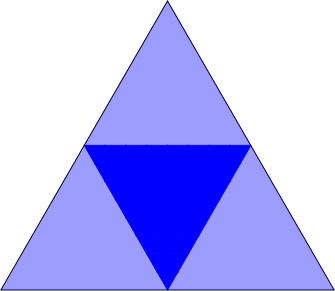Next largest shaded triangle: occupies $\dfrac14$ of $\frac14$ $\therefore$ area $=\frac1{16}$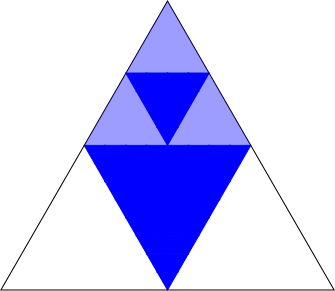That means the shaded triangles of this size occupy $3\times\dfrac1{16}=\dfrac3{16}$ of the whole triangle.

Next smallest shaded triangles:  $\dfrac14$ of $\dfrac1{16}=\dfrac1{64}$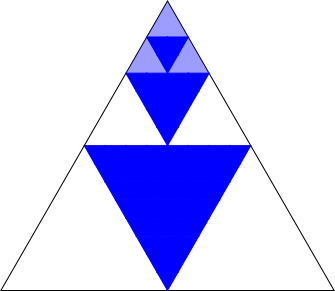That means the shaded triangles of this size occupy $3\times\dfrac1{16}=\dfrac3{16}$ of the whole triangle.

Smallest shaded triangles: $\dfrac14\times\dfrac1{64}=\dfrac1{256}$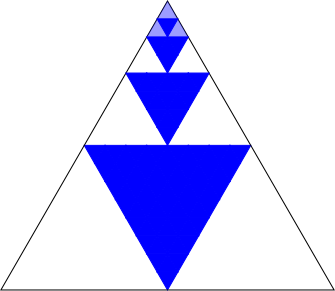That means the shaded triangles of this size occupy $3\times\dfrac1{256}=\dfrac3{256}$ of the whole triangle.

So the total shaded area is $\dfrac14+\dfrac3{16}+\dfrac3{64}+\dfrac{3}{256}$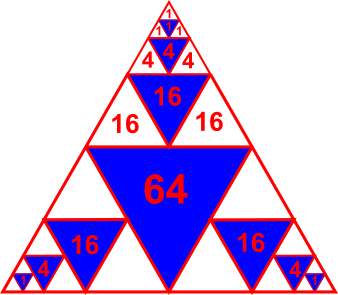$=\dfrac{64+3\times16+3\times4+3\times1}{256}\\ =\dfrac{127}{256}$

You can find more short problems, arranged by curriculum topic, in our short problems collection.